### Crack propagation

In CalculiX a rather simple model to calculate cyclic crack propagation is implemented. In order to perform a crack propagation calculation the following procedure is to be followed:

• A static calculation (usually called a Low Cycle Fatigue = LCF calculation) for the uncracked structure (using volumetric elements) for one or more steps must have been performed and the results (at least stresses; if applicable, also the temperatures) must have been stored in a frd-file.
• Optionally a frequency calculation (usually called a High Cycle Fatigue = HCF calculation) for the uncracked structure has been performed and the results (usually stresses) have been stored in a frd-file.
• For the crack propagation itself a model consisting of at least all cracks to be considered meshed using S3-shell elements must be created. The orientation of all shell elements used to model one and the same crack should consistent, i.e. when viewing the crack from one side of the crack shape all nodes should be numbered clockwise or all nodes should be numbered counterclockwise. Preferably, also the mesh of the uncracked structure should be contained (the crack propagation can be easier interpreted if the structure in which the crack propagates is also visualized) .
• The material parameters for the crack propagation law implemented in CalculiX must have been determined. Alternatively, the user may code his/her own crack propagation law in routine crackrate.f.
• The procedure *CRACK PROPAGATION must have been selected with appropriate parameters. Within the *CRACK PROPAGATION step the optional keyword card *HCF may have been selected.

In CalculiX, the following crack propagation law has been implemented: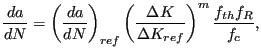(578)

where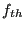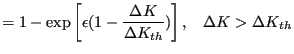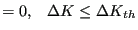(579)

accounts for the threshold range,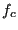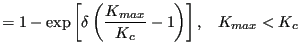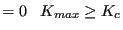(580)

for the critical cut-off and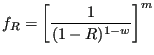(581)

for the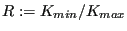influence. The material constants have to be entered by using a *USER MATERIAL card with the following 8 constants per temperature data point (in that order):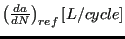,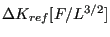,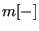,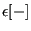,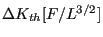,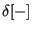,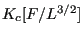and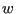[-], were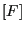is the unit of force and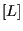of length. Notice that the first part of the law corresponds to the Paris law. Indeed the classical Paris constant C can be obtained from: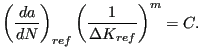(582)

Vice versa,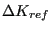can be obtained from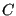using the above equation once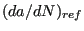has been chosen. Notice thatis the rate for which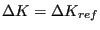(just considering the Paris range). For a user material, a maximum of 8 constants can be defined per line (cf. *USER MATERIAL). Therefore, after entering the 8 crack propagation constants, the corresponding temperature has to be entered on a new line.

The crack propagation calculation consists of a number of increments during which the crack propagates a certain amount. For each increment in a LCF calculation the following steps are performed:

• The actual shape of the cracks is analyzed, the crack fronts are determined and the stresses and temperatures (if applicable, else zero) at the crack front nodes are interpolated from the stress and temperature field in the uncracked structure.
• The stress tensor at the front nodes is projected on the local tangent plane yielding a normal component (local y-direction), a shear component orthogonal to the crack front (local x-direction) and one parallel to the crack front (local z-direction), leading to the K-factors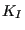,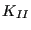and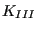using the formulas: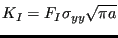(583)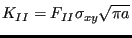(584)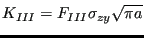(585)

where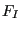,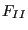and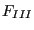are shape factors taking the form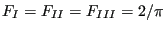(586)

for subsurface cracks,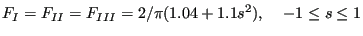(587)

for surface cracks spanning an angle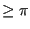and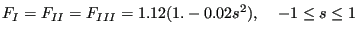(588)

for surface cracks spanning an angle of 0 (i.e. a one-sided crack in a two-dimensional plate). For an angle in between 0 and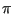the shape factors are linearly interpolated in between the latter two formulas. In the above formulas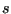is a local coordinate along the crack front, taking the values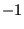and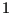at the free surface and 0 in the middle of the front. If the user prefers to use more detailed shape factors, user routine crackshape.f can be recoded.

• The crack length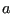in the above formulas is determined in two different ways, depending on the value of the parameter LENGTH on the *CRACK PROPAGATION card:

• for LENGTH=CUMULATIVE the crack length is obtained by incrementally adding the crack propagation increments to the initial crack length. The initial length is determined using the LENGTH=INTERSECTION method.
• for LENGTH=INTERSECTION a plane locally orthogonal to the crack front is constructed and subsequently a second intersection of this plane with the crack front is sought. The distance in between these intersection points is the crack length (except for a subsurface crack for which this length is divided by two). Notice that for intersection purposes the crack front for a surface crack is artificially closed by the intersection curve of the crack shape with the free surface in between the intersection points of the crack front.

Subsequently, the crack length is smoothed along the crack front according to: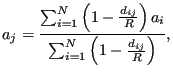(589)

where the sum is over the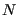closest nodes,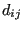is the Euclidean incremental distance between node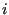and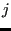, and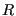is the distance between nodeand the farthest of these nodes.is a fixed fraction of the total number of nodes along the front, e.g. 90 %.

• From the stress factors an equivalent K-factor and deflection angle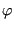is calculated using a light modification of the formulas by Richard  in order to cope with negativevalues as well: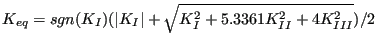(590)

and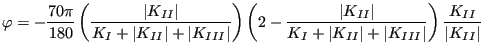(591)

for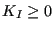and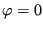else. Subsequenty,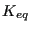andare smoothed in the same way as the crack length. Finally, if any of the deflection angles exceeds the maximum defined by the user (second entry underneath the *CRACK PROPAGATION card) all values along the front are scaled appropriately.

Notice that at each crack front location as manyandvalues are calculated as there are steps in the static calculation of the uncracked structure.

• The crack propagation increment for this increment is determined. It is the minimum of:

• The user defined value (first entry underneath the *CRACK PROPAGATION card)
• one fifth of the minimum crack front curvature
• one fifth of the smallest crack length

• The crack propagation rate at every crack front location is determined. If there is only one step it results from the direct application of the crack propagation law with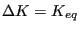. For several steps the maximum minus the minimum ofis taken. Notice that the crack rate routine is documented as a user subroutine: for missions consisting of several steps the user can define his/her own procedure for more complex procedures such as cycle extraction. The maximum value of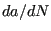across all crack front locations determines the number of cycles in this increment.

• For each crack front node the location of the propagated node is determined. This node lies in a plane locally orthogonal to the tangent vector along the front. To this end a local coordinate system is created (the same as for the calculation of,and) consisting of:

• The local tangent vector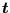.
• The local normal vector obtained by the mean of the normal vectors on the shell elements to which the nodal front position belongs. This vector is subsequently projected into the plane normal toand normalized to obtain a vector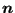.
• a vector in the propagation direction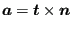. This assumes that the tangent vector was such that the corkscrew rule points into directionwhen running along the crack front in direction.

• Then, new nodes are created in between the propagated nodes such that they are equidistant. The target distance in between these nodes is the mean distance in between the nodes along the initial crack front.

• Finally, new shell elements are generated covering the crack propagation increment and the results (K-values, crack length etc.) are stored in frd-format for visualization. Then, a new increment can start. The number of increments is governed by the INC parameter on the *STEP card.

For a combined LCF-HCF calculation, triggered by the *HCF keyword in the *CRACK PROPAGATION procedure the picture is slightly more complicated. On the *HCF card the user defines a scaling factor and a step from the static calculation on which the HCF loading is to be applied. This is usually the static loading at which the modal excitation occurs. At this step a HCF cycle is considered consisting of the LCF+HCF and the LCF-HCF loading. The effect is as follows:

• If this cycle leads to propagaton and HCF propagation is not allowed (MAX CYCLE= 0 on the *HCF card; this is default) the program stops with an appropriate error message.
• If it leads to propagation and HCF propagation is allowed (MAX CYCLE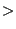0 on the *HCF card) the number of cycles is determined to reach the desired crack propagation in this increment and the next increment is started. No LCF propagation is considered in this increment.
• If it does not lead to HCF propagation, LCF propagation is considered for the static loading in which the LCF loading of the step to which HCF applies is repaced by LCF+HCF loading. The propagation is calculated as usual.

Right now, the output of a *CRACK PROPAGATION step cannot be influenced by the user. By default a data set is created in the frd-file consisting of the following information (most of this information can be changed in user subroutine crackrate.f):

• The dominant step. This is the step with the largest(over all steps).
• DeltaKEQ: the value of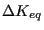for the main cycle. In the present implementation this corresponds to the largest value of(over all steps).
• KEQMIN: the minimal value of(over all steps).
• KEQMAX: the largest value of(over all steps).
• K1WORST: the largest value of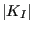multiplied by its sign (over all steps).
• K2WORST: the largest value of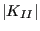multiplied by its sign (over all steps).
• K3WORST: the largest value of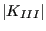multiplied by its sign (over all steps).
• PHI: the deflection angle.
• R: the R-value of the main cycle. In the present implementation this is zero.
• DADN: the crack propagation rate.
• KTH: not used.
• INC: the increment number. This is the same for all nodes along one and the same crack front.
• CYCLES: the number of cycles since the start of the calculation. This number is common to all crack front nodes.
• CRLENGTH: crack length.
• DOM_SLIP: not used